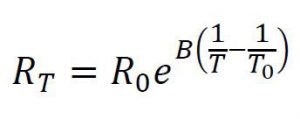# What is thermistor?

Thermistor is short form of thermal resistor and it is thermally sensitive resistor. A thermistor is a temperature-sensing element composed of sintered semiconductor material which exhibits a large change in resistance proportional to a small change in temperature.

## Construction:

The thermistor is made up of ceramic like semi-conducting materials such as copper oxide, manganese oxide, nickel oxide, cobalt oxide, titanium oxide and lithium oxide. These oxides are blended in a suitable proportion and compressed into the desired shapes from the mixed powers and heat treated to recrystallise them, resulting in a dense ceramic body with the required resistance temperature characteristics.

## Working:

As the temperature increases, its resistance decreases and vice versa and this is called as negative temperature characteristic of thermocouple. The thermistor is a very non linear instrument where as resistors such as platinum, copper and tungsten are quite linear. The resistance temperature relation for thermistor is given below.Where,

R = Resistance at any temperature (T).

R0 = Resistance at the reference temperature (T0).

β = 3000 K to 4500 K depending on its composition.

The practical operating range of thermistor lies between -100 to +300 Celsius. Normally thermistor shows NTC characteristics but some specially designed shows PTC characteristics up to some specified range.